IM10. Other geometries

Previously, we looked at a few common geometries of carbon atoms in molecules. Carbon most often has four neighbouring atoms in the molecule, and in that case it is tetrahedral. However, sometimes there are only two or three neighboring atoms, and so linear or trigonal planar geometries are also pretty common.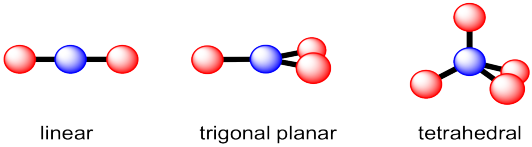These geometries are found throughout the periodic table for atoms that have 2, 3 or 4 neighboring atoms within a molecule. However, there are also some other molecular geometries that are pretty common.

Earlier, we looked at the environments of atoms or ions within solid structures and considered the "coordination number" of the atom or ion. Remember, the coordination number is just the number of atoms in close contact with a central atom. We can think about the geometry around a particular atom in a molecule the same way.

Coordination number 4

There are actually two different geometric options that occur with some regularity for coordination number 4. Tetrahedral geometry, which we have seen, maximizes the distances between neighbours. That could be advantageous because it minimizes crowding. In fact, tetrahedral geometry is the most common geometry for tjis coordination number. However, a second geometry is available for a limited number of compounds. In square planar geometry, all four neighbours are found in the same plane and are roughly 90 degrees apart from each other. This geometry is most often observed for certain compounds of palladium and platinum as well as a few other transition metals.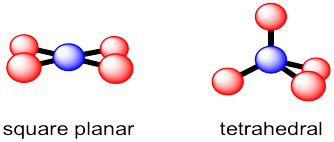Coordination number 5

In the case of five neighboring atoms, there are two geometries that are roughly equally prevalent. In a trigonal bipyramidal structure, the central atom is surrounded by three neighbours that form a trigonal plane around it. The last two neighbours are above and below this plane. These two neighbours are roughly colinear with the central atom. The trigonal planar positions referred to as "equatorial;" the other two positions are called "axial."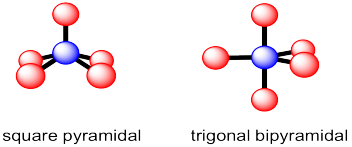The other common five coordinate geometry is square pyramidal. In this case, the central atom sits above a base of four neighbors; those four positions form a square plane together. A fifth atom occupies the space above the central atom.

Coordination number 6

Almost all examples of atoms with coordination number six can be described as having an octahedral geometry. Four of the neighbours form a square plane around the central atom, with one atom above this plane and one below it. As with the trigonal bipyramid, these positions can be described as equatorial and axial, respectively.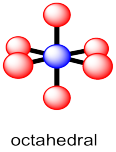Electronic vs. molecular geometry

There is a striking difference between geometries around transition metals, in the middle of the periodic table, compared to main group p-block elements, in the right hand part of the periodic table. The geometry around a transition metal is simply based on the number of atoms bonded to it. Each surrounding atom takes up some space and so a geometry is adopted to give those atoms roughly equal space around the central atom.

In the p-block, things are generally quite different. It's not just the neighbouring atoms that take up space; the valence electrons on the central atom also have to be accommodated. Thus, in ammonia, NH3, we need to consider not just the three hydrogen atoms that are bonded to the nitrogen, but nitrogen's own lone pair as well, in order to determine the geometry. That's four objects to fit around the nitrogen atom. The normal geometry for four things is tetrahedral. Thus, ammonia takes on a tetrahedral shape. It isn't trigonal planar.

That's sometimes called the overall electronic geometry. It is the geometry of everything, including lone pair electrons. To see the relationship between the atoms -- the nitrogen and its three nieghbouring hydrogens -- just ignore the electron pair for a minute. The nitrogen sits above a trigonal base of three hydrogen atoms. The molecular geometry -- that shape formed by the nitrogen and the three hydrogens -- is pyramidal.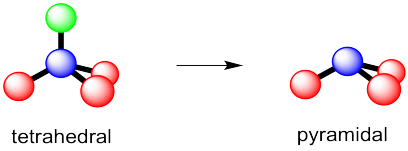This phenomenon, in which the geometric relationship between atoms is influenced by where the electrons are on a central atom, is pretty common around chalcogens and pnictogens -- that is, oxygen and the other atoms in its column of the periodic table, as well as nitrogen and the other atoms in its group. In those cases, lone pairs appear to force the surrounding atoms a little closer to each other.

Other than that, compounds with different electronic versus molecular geometry are pretty rare in terms of numbers of examples, although those few examples do illustrate a wide variety of geometries.

Problem IM10.1.

Suppose a compound had a central atom surrounded by six groups, including bonds to other atoms as well as its own lone pairs.

1. What is its overall "electronic" geometry?
2. What would be its molecular geometry if it had one lone pair?
3. What would be its molecular geometry if it had two lone pairs?

In cases where the molecular geometry is different from the electronic geometry, we sometimes need to make choices about which position a lone pair is more likely to occupy. The general rule is that lone pairs occupy the less crowded site. It is as if the lone pair takes up more space than a bond to an atom.

Problem IM10.2.

Suppose a compound had a central atom surrounded by five groups, including bonds to other atoms as well as its own lone pairs.

1. What is its overall "electronic" geometry?
2. What would be its molecular geometry if it had one lone pair?
3. What would be its molecular geometry if it had two lone pairs?

Problem IM10.3.

Draw structures for the following compounds.

a) H2O b) (CH3)3O+ c) PCl3 d) SF4 e) ClF3 f) PCl5

g) SF6 h) ClF5 i) XeF4

Problem IM10.4.

What is the molecular geometry of each of the compounds in problem IM10.3?